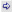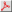BACK TO INDEX BACK TO OTHMAR FREY'S HOMEPAGE

## Publications of year 1951

### Articles in journal or book chapters

1. L. Essen and K. D. Froome. The Refractive Indices and Dielectric Constants of Air and its Principal Constituents at 24,000 Mc/s. Proceedings of the Physical Society. Section B, 64(10):862, 1951.Abstract: The refractive indices of air and its principal constituents have been measured at a frequency of 24000 Mc/s. with a precision comparable with that obtained in the optical range. The method is based on the measurement of the resonant frequency of a cavity resonator first when it is filled with the gas and then when it is evacuated. The source of oscillations used is a Pound-stabilized velocity-modulated oscillator, and its frequency is measured by reference to a high harmonic of a quartz standard, with a precision of 1 part in 10^8 . The cavity is provided with a tuning plunger, which changes the resonant frequency through a range of about 10 Mc/s, and is calibrated to an accuracy of within 1 kc/s. Most of the measurements were made by the frequency-change method and the plunger was used in a narrow region only for a precise setting to resonance. It is possible by a larger movement of the plunger to compensate for the whole frequency change due to the removal of the gas and thus to work at a fixed frequency. The following results were obtained for ( n - 1)10 6 where n is the refractive index at 0 deg C., 760 mm Hg: dry CO2 - free air, 288.15 +/- 0.1; nitrogen, 294.1 +/-0 1; oxygen, 266.4 +/-0.2; argon, 277.8 +/- 0.2; carbon dioxide, 494 +/- 1; and the value for water vapour at 20 deg C, 10 mm. Hg pressure was 60.7 +/- 0.1. The dielectric constants can be calculated from the relationship mu epsilon = n^2 , where mu is the magnetic permeability and epsilon is the dielectric constant, the values of (mu - 1) 10^6 being taken as 0.4 for air, 1.9 for oxygen and zero for the other gases. Accurate formulae are given for obtaining the refractive index of moist air at different atmospheric conditions, and are reduced to the following simple formula which is applicable for normal atmospheric conditions: (n_t,p - 1)*10^6 = (103.49/T)*p_1 + (177.4/T)*p_2 + (86.26/T)(1+5748/T)*p_3, where p_1, p_2, p_3 are the partial pressures of dry air, carbon dioxide and water vapour, t is the temperature in degrees C., and T = 273 + t is the absolute temperature. A value of 1.839+/-0.002 * 10^-18 E S.U. was derived for the dipole moment of the water vapour molecule.

```@Article{essenFroome1951RefractiveIndexAir,
author = {L. Essen and K. D. Froome},
title = {The Refractive Indices and Dielectric Constants of Air and its Principal Constituents at 24,000 Mc/s},
journal = {Proceedings of the Physical Society. Section B},
year = {1951},
volume = {64},
number = {10},
pages = {862},
abstract = {The refractive indices of air and its principal constituents have been measured at a frequency of 24000 Mc/s. with a precision comparable with that obtained in the optical range. The method is based on the measurement of the resonant frequency of a cavity resonator first when it is filled with the gas and then when it is evacuated. The source of oscillations used is a Pound-stabilized velocity-modulated oscillator, and its frequency is measured by reference to a high harmonic of a quartz standard, with a precision of 1 part in 10^8 . The cavity is provided with a tuning plunger, which changes the resonant frequency through a range of about 10 Mc/s, and is calibrated to an accuracy of within 1 kc/s. Most of the measurements were made by the frequency-change method and the plunger was used in a narrow region only for a precise setting to resonance. It is possible by a larger movement of the plunger to compensate for the whole frequency change due to the removal of the gas and thus to work at a fixed frequency. The following results were obtained for ( n - 1)10 6 where n is the refractive index at 0 deg C., 760 mm Hg: dry CO2 - free air, 288.15 +/- 0.1; nitrogen, 294.1 +/-0 1; oxygen, 266.4 +/-0.2; argon, 277.8 +/- 0.2; carbon dioxide, 494 +/- 1; and the value for water vapour at 20 deg C, 10 mm. Hg pressure was 60.7 +/- 0.1. The dielectric constants can be calculated from the relationship mu epsilon = n^2 , where mu is the magnetic permeability and epsilon is the dielectric constant, the values of (mu - 1) 10^6 being taken as 0.4 for air, 1.9 for oxygen and zero for the other gases. Accurate formulae are given for obtaining the refractive index of moist air at different atmospheric conditions, and are reduced to the following simple formula which is applicable for normal atmospheric conditions: (n_t,p - 1)*10^6 = (103.49/T)*p_1 + (177.4/T)*p_2 + (86.26/T)(1+5748/T)*p_3, where p_1, p_2, p_3 are the partial pressures of dry air, carbon dioxide and water vapour, t is the temperature in degrees C., and T = 273 + t is the absolute temperature. A value of 1.839+/-0.002 * 10^-18 E S.U. was derived for the dipole moment of the water vapour molecule.},
file = {:essenFroome1951RefractiveIndexAir.pdf:PDF},
pdf = {essenFroome1951RefractiveIndexAir.pdf},
url = {http://stacks.iop.org/0370-1301/64/i=10/a=303},

}
```

BACK TO INDEX BACK TO OTHMAR FREY'S HOMEPAGE

Disclaimer:

Please note that access to full text PDF versions of papers is restricted to the Chair of Earth Observation and Remote Sensing, Institute of Environmental Engineering, ETH Zurich.
Copyright and all rights therein are retained by authors or by other copyright holders. All persons copying this information are expected to adhere to the terms and constraints invoked by each author's copyright.

This collection of SAR literature is far from being complete.
It is rather a collection of papers which I store in my literature data base. Hence, the list of publications under PUBLICATIONS OF AUTHOR'S NAME should NOT be mistaken for a complete bibliography of that author.